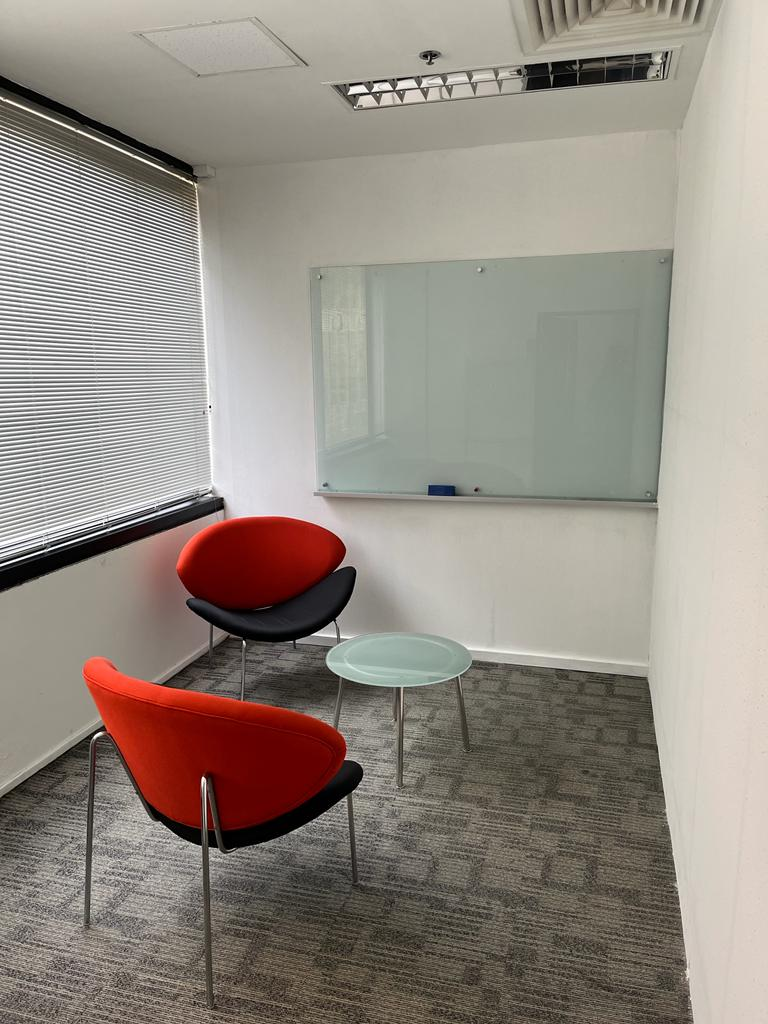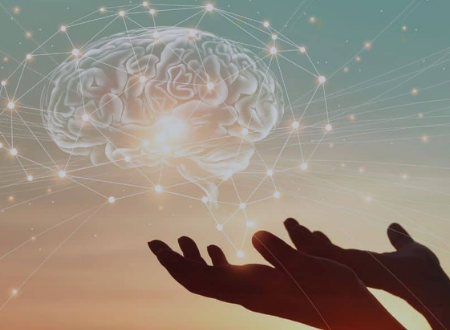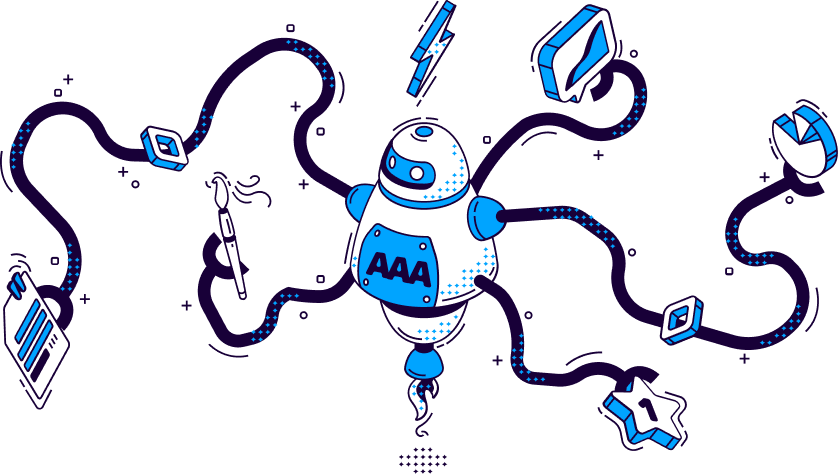# Aventis Centre for Wellness & Organisational Psychology

WHAT WE DO?

At Aventis Centre for Wellness & Organisational Psychology, we recognise the need of better mental well-being. We are here to support you and all companies towards a healthier and more engaged workforce by providing workplace well-being solutions.

We focus on keeping your employees and you happy, engaged, and healthy through our 3 core focuses - EAP Organizational Counselling, Individual Counselling and Bespoke Wellness Programmes

```!function(t,n){"object"==typeof exports&&"undefined"!=typeof module?module.exports=n():"function"==typeof define&&define.amd?define(n):(t="undefined"!=typeof globalThis?globalThis:t||self).__SVGATOR_PLAYER__=n()}(this,(function(){"use strict";function t(n){return(t="function"==typeof Symbol&&"symbol"==typeof Symbol.iterator?function(t){return typeof t}:function(t){return t&&"function"==typeof Symbol&&t.constructor===Symbol&&t!==Symbol.prototype?"symbol":typeof t})(n)}function n(t,n){if(!(t instanceof n))throw new TypeError("Cannot call a class as a function")}function r(t,n){for(var r=0;r<n.length;r++){var e=n[r];e.enumerable=e.enumerable||!1,e.configurable=!0,"value"in e&&(e.writable=!0),Object.defineProperty(t,e.key,e)}}function e(t,n,e){return n&&r(t.prototype,n),e&&r(t,e),t}function i(t){return(i=Object.setPrototypeOf?Object.getPrototypeOf:function(t){return t.__proto__||Object.getPrototypeOf(t)})(t)}function o(t,n){return(o=Object.setPrototypeOf||function(t,n){return t.__proto__=n,t})(t,n)}function u(t,n){return!n||"object"!=typeof n&&"function"!=typeof n?function(t){if(void 0===t)throw new ReferenceError("this hasn't been initialised - super() hasn't been called");return t}(t):n}function a(t){var n=function(){if("undefined"==typeof Reflect||!Reflect.construct)return!1;if(Reflect.construct.sham)return!1;if("function"==typeof Proxy)return!0;try{return Boolean.prototype.valueOf.call(Reflect.construct(Boolean,[],(function(){}))),!0}catch(t){return!1}}();return function(){var r,e=i(t);if(n){var o=i(this).constructor;r=Reflect.construct(e,arguments,o)}else r=e.apply(this,arguments);return u(this,r)}}function l(t,n,r){return(l="undefined"!=typeof Reflect&&Reflect.get?Reflect.get:function(t,n,r){var e=function(t,n){for(;!Object.prototype.hasOwnProperty.call(t,n)&&null!==(t=i(t)););return t}(t,n);if(e){var o=Object.getOwnPropertyDescriptor(e,n);return o.get?o.get.call(r):o.value}})(t,n,r||t)}var f=s(Math.pow(10,-6));function s(t){var n=arguments.length>1&&void 0!==arguments?arguments:6;if(Number.isInteger(t))return t;var r=Math.pow(10,n);return Math.round((+t+Number.EPSILON)*r)/r}function c(t,n){var r=arguments.length>2&&void 0!==arguments?arguments:f;return Math.abs(t-n)<r}var h=Math.PI/180;function v(t){return t}function y(t,n,r){var e=1-r;return 3*r*e*(t*e+n*r)+r*r*r}function d(){var t=arguments.length>0&&void 0!==arguments?arguments:0,n=arguments.length>1&&void 0!==arguments?arguments:0,r=arguments.length>2&&void 0!==arguments?arguments:1,e=arguments.length>3&&void 0!==arguments?arguments:1;return t<0||t>1||r<0||r>1?null:c(t,n)&&c(r,e)?v:function(i){if(i<=0)return t>0?i*n/t:0===n&&r>0?i*e/r:0;if(i>=1)return r<1?1+(i-1)*(e-1)/(r-1):1===r&&t<1?1+(i-1)*(n-1)/(t-1):1;for(var o,u=0,a=1;u<a;){var l=y(t,r,o=(u+a)/2);if(c(i,l))break;l<i?u=o:a=o}return y(n,e,o)}}function g(){return 1}function p(t){return 1===t?1:0}function m(){var t=arguments.length>0&&void 0!==arguments?arguments:1,n=arguments.length>1&&void 0!==arguments?arguments:0;if(1===t){if(0===n)return p;if(1===n)return g}var r=1/t;return function(t){return t>=1?1:(t+=n*r)-t%r}}function b(t,n,r){return t>=.5?r:n}function w(t,n,r){return 0===t||n===r?n:t*(r-n)+n}function x(t,n,r){var e=w(t,n,r);return e<=0?0:e}function k(t,n,r){return 0===t?n:1===t?r:{x:w(t,n.x,r.x),y:w(t,n.y,r.y)}}function A(t,n,r){var e=function(t,n,r){return Math.round(w(t,n,r))}(t,n,r);return e<=0?0:e>=255?255:e}function S(t,n,r){return 0===t?n:1===t?r:{r:A(t,n.r,r.r),g:A(t,n.g,r.g),b:A(t,n.b,r.b),a:w(t,null==n.a?1:n.a,null==r.a?1:r.a)}}function _(t,n,r){if(0===t)return n;if(1===t)return r;var e=n.length;if(e!==r.length)return b(t,n,r);for(var i=[],o=0;o<e;o++)i.push(S(t,n[o],r[o]));return i}function O(t,n){for(var r=[],e=0;e<t;e++)r.push(n);return r}function M(t,n){if(--n<=0)return t;var r=(t=Object.assign([],t)).length;do{for(var e=0;e<r;e++)t.push(t[e])}while(--n>0);return t}var j,P=function(){function t(r){n(this,t),this.list=r,this.length=r.length}return e(t,[{key:"setAttribute",value:function(t,n){for(var r=this.list,e=0;e<this.length;e++)r[e].setAttribute(t,n)}},{key:"removeAttribute",value:function(t){for(var n=this.list,r=0;r<this.length;r++)n[r].removeAttribute(t)}},{key:"style",value:function(t,n){for(var r=this.list,e=0;e<this.length;e++)r[e].style[t]=n}}]),t}(),I=/-./g,E=function(t,n){return n.toUpperCase()};function F(t){return"function"==typeof t?t:b}function N(t){return t?"function"==typeof t?t:Array.isArray(t)?function(t){var n=arguments.length>1&&void 0!==arguments?arguments:v;if(!Array.isArray(t))return n;switch(t.length){case 1:return m(t)||n;case 2:return m(t,t)||n;case 4:return d(t,t,t,t)||n}return n}(t,null):function(t,n){var r=arguments.length>2&&void 0!==arguments?arguments:v;switch(t){case"linear":return v;case"steps":return m(n.steps||1,n.jump||0)||r;case"bezier":case"cubic-bezier":return d(n.x1||0,n.y1||0,n.x2||0,n.y2||0)||r}return r}(t.type,t.value,null):null}function B(t,n,r){var e=arguments.length>3&&void 0!==arguments&&arguments,i=n.length-1;if(t<=n.t)return e?[0,0,n.v]:n.v;if(t>=n[i].t)return e?[i,1,n[i].v]:n[i].v;var o,u=n,a=null;for(o=1;o<=i;o++){if(!(t>n[o].t)){a=n[o];break}u=n[o]}return null==a?e?[i,1,n[i].v]:n[i].v:u.t===a.t?e?[o,1,a.v]:a.v:(t=(t-u.t)/(a.t-u.t),u.e&&(t=u.e(t)),e?[o,t,r(t,u.v,a.v)]:r(t,u.v,a.v))}function R(t,n){var r=arguments.length>2&&void 0!==arguments?arguments:null;return t&&t.length?"function"!=typeof n?null:("function"!=typeof r&&(r=null),function(e){var i=B(e,t,n);return null!=i&&r&&(i=r(i)),i}):null}function q(t,n){return t.t-n.t}function T(n,r,e,i,o){var u,a="@"===e,l="#"===e,f=j[e],s=b;switch(a?(u=e.substr(1),e=u.replace(I,E)):l&&(e=e.substr(1)),t(f)){case"function":if(s=f(i,o,B,N,e,a,r,n),l)return s;break;case"string":s=R(i,F(f));break;case"object":if((s=R(i,F(f.i),f.f))&&"function"==typeof f.u)return f.u(r,s,e,a,n)}return s?function(t,n,r){var e=arguments.length>3&&void 0!==arguments&&arguments;if(e)return t instanceof P?function(e){return t.style(n,r(e))}:function(e){return t.style[n]=r(e)};if(Array.isArray(n)){var i=n.length;return function(e){var o=r(e);if(null==o)for(var u=0;u<i;u++)t[u].removeAttribute(n);else for(var a=0;a<i;a++)t[a].setAttribute(n,o)}}return function(e){var i=r(e);null==i?t.removeAttribute(n):t.setAttribute(n,i)}}(r,e,s,a):null}function L(n,r,e,i){if(!i||"object"!==t(i))return null;var o=null,u=null;return Array.isArray(i)?u=function(t){if(!t||!t.length)return null;for(var n=0;n<t.length;n++)t[n].e&&(t[n].e=N(t[n].e));return t.sort(q)}(i):(u=i.keys,o=i.data||null),u?T(n,r,e,u,o):null}function C(t,n,r){if(!r)return null;var e=[];for(var i in r)if(r.hasOwnProperty(i)){var o=L(t,n,i,r[i]);o&&e.push(o)}return e.length?e:null}function z(t,n){if(!n.duration||n.duration<0)return null;var r=function(t,n){if(!n)return null;var r=[];if(Array.isArray(n))for(var e=n.length,i=0;i<e;i++){var o=n[i];if(2===o.length){var u=null;if("string"==typeof o)u=t.getElementById(o);else if(Array.isArray(o)){u=[];for(var a=0;a<o.length;a++)if("string"==typeof o[a]){var l=t.getElementById(o[a]);l&&u.push(l)}u=u.length?1===u.length?u:new P(u):null}if(u){var f=C(t,u,o);f&&(r=r.concat(f))}}}else for(var s in n)if(n.hasOwnProperty(s)){var c=t.getElementById(s);if(c){var h=C(t,c,n[s]);h&&(r=r.concat(h))}}return r.length?r:null}(t,n.elements);return r?function(t,n){var r=arguments.length>2&&void 0!==arguments?arguments:1/0,e=arguments.length>3&&void 0!==arguments?arguments:1,i=arguments.length>4&&void 0!==arguments&&arguments,o=arguments.length>5&&void 0!==arguments?arguments:1,u=t.length,a=e>0?n:0;i&&r%2==0&&(a=n-a);var l=null;return function(f,s){var c=f%n,h=1+(f-c)/n;s*=e,i&&h%2==0&&(s=-s);var v=!1;if(h>r)c=a,v=!0,-1===o&&(c=e>0?0:n);else if(s<0&&(c=n-c),c===l)return!1;l=c;for(var y=0;y<u;y++)t[y](c);return v}}(r,n.duration,n.iterations||1/0,n.direction||1,!!n.alternate,n.fill||1):null}Number.isInteger||(Number.isInteger=function(t){return"number"==typeof t&&isFinite(t)&&Math.floor(t)===t}),Number.EPSILON||(Number.EPSILON=2220446049250313e-31);var D=function(){function t(r,e){var i=arguments.length>2&&void 0!==arguments?arguments:{};n(this,t),this._id=0,this._running=!1,this._rollingBack=!1,this._animations=r,this.duration=e.duration,this.alternate=e.alternate,this.fill=e.fill,this.iterations=e.iterations,this.direction=i.direction||1,this.speed=i.speed||1,this.fps=i.fps||100,this.offset=i.offset||0,this.rollbackStartOffset=0}return e(t,[{key:"_rollback",value:function(){var t=this,n=1/0,r=null;this.rollbackStartOffset=this.offset,this._rollingBack||(this._rollingBack=!0,this._running=!0);this._id=window.requestAnimationFrame((function e(i){if(t._rollingBack){null==r&&(r=i);var o=i-r,u=t.rollbackStartOffset-o,a=Math.round(u*t.speed);if(a>t.duration&&n!=1/0){var l=!!t.alternate&&a/t.duration%2>1,f=a%t.duration;a=(f+=l?t.duration:0)||t.duration}var s=t.fps?1e3/t.fps:0,c=Math.max(0,a);if(c<n-s){t.offset=c,n=c;for(var h=t._animations,v=h.length,y=0;y<v;y++)h[y](c,t.direction)}var d=!1;if(t.iterations>0&&-1===t.fill){var g=t.iterations*t.duration,p=g==a;a=p?0:a,t.offset=p?0:t.offset,d=a>g}a>0&&t.offset>=a&&!d?t._id=window.requestAnimationFrame(e):t.stop()}}))}},{key:"_start",value:function(){var t=this,n=arguments.length>0&&void 0!==arguments?arguments:0,r=-1/0,e=null,i={},o=function o(u){t._running=!0,null==e&&(e=u);var a=Math.round((u-e+n)*t.speed),l=t.fps?1e3/t.fps:0;if(a>r+l&&!t._rollingBack){t.offset=a,r=a;for(var f=t._animations,s=f.length,c=0,h=0;h<s;h++)i[h]?c++:(i[h]=f[h](a,t.direction),i[h]&&c++);if(c===s)return void t._stop()}t._id=window.requestAnimationFrame(o)};this._id=window.requestAnimationFrame(o)}},{key:"_stop",value:function(){this._id&&window.cancelAnimationFrame(this._id),this._running=!1,this._rollingBack=!1}},{key:"play",value:function(){!this._rollingBack&&this._running||(this._rollingBack=!1,this.rollbackStartOffset>this.duration&&(this.offset=this.rollbackStartOffset-(this.rollbackStartOffset-this.offset)%this.duration,this.rollbackStartOffset=0),this._start(this.offset))}},{key:"stop",value:function(){this._stop(),this.offset=0,this.rollbackStartOffset=0;var t=this.direction,n=this._animations;window.requestAnimationFrame((function(){for(var r=0;r<n.length;r++)n[r](0,t)}))}},{key:"reachedToEnd",value:function(){return this.iterations>0&&this.offset>=this.iterations*this.duration}},{key:"restart",value:function(){this._stop(),this.offset=0,this._start()}},{key:"pause",value:function(){this._stop()}},{key:"reverse",value:function(){this.direction=-this.direction}}],[{key:"build",value:function(n,r){return(n=function(t,n){if(j=n,!t||!t.root||!Array.isArray(t.animations))return null;for(var r=document.getElementsByTagName("svg"),e=!1,i=0;i<r.length;i++)if(r[i].id===t.root&&!r[i].svgatorAnimation){(e=r[i]).svgatorAnimation=!0;break}if(!e)return null;var o=t.animations.map((function(t){return z(e,t)})).filter((function(t){return!!t}));return o.length?{element:e,animations:o,animationSettings:t.animationSettings,options:t.options||void 0}:null}(n,r))?{el:n.element,options:n.options||{},player:new t(n.animations,n.animationSettings,n.options)}:null}}]),t}();function Q(t){return s(t)+""}function U(t){var n=arguments.length>1&&void 0!==arguments?arguments:" ";return t&&t.length?t.map(Q).join(n):""}function V(t){return t?null==t.a||t.a>=1?"rgb("+t.r+","+t.g+","+t.b+")":"rgba("+t.r+","+t.g+","+t.b+","+t.a+")":"transparent"}!function(){for(var t=0,n=["ms","moz","webkit","o"],r=0;r<n.length&&!window.requestAnimationFrame;++r)window.requestAnimationFrame=window[n[r]+"RequestAnimationFrame"],window.cancelAnimationFrame=window[n[r]+"CancelAnimationFrame"]||window[n[r]+"CancelRequestAnimationFrame"];window.requestAnimationFrame||(window.requestAnimationFrame=function(n){var r=Date.now(),e=Math.max(0,16-(r-t)),i=window.setTimeout((function(){n(r+e)}),e);return t=r+e,i},window.cancelAnimationFrame=window.clearTimeout)}();var G={f:null,i:function(t,n,r){return 0===t?n:1===t?r:{x:x(t,n.x,r.x),y:x(t,n.y,r.y)}},u:function(t,n){return function(r){var e=n(r);t.setAttribute("rx",Q(e.x)),t.setAttribute("ry",Q(e.y))}}},H={f:null,i:function(t,n,r){return 0===t?n:1===t?r:{width:x(t,n.width,r.width),height:x(t,n.height,r.height)}},u:function(t,n){return function(r){var e=n(r);t.setAttribute("width",Q(e.width)),t.setAttribute("height",Q(e.height))}}},Y=Math.sin,Z=Math.cos,J=Math.acos,K=Math.asin,W=Math.tan,X=Math.atan2,\$=Math.PI/180,tt=180/Math.PI,nt=Math.sqrt,rt=function(){function t(){var r=arguments.length>0&&void 0!==arguments?arguments:1,e=arguments.length>1&&void 0!==arguments?arguments:0,i=arguments.length>2&&void 0!==arguments?arguments:0,o=arguments.length>3&&void 0!==arguments?arguments:1,u=arguments.length>4&&void 0!==arguments?arguments:0,a=arguments.length>5&&void 0!==arguments?arguments:0;n(this,t),this.m=[r,e,i,o,u,a],this.i=null,this.w=null,this.s=null}return e(t,[{key:"determinant",get:function(){var t=this.m;return t*t-t*t}},{key:"isIdentity",get:function(){if(null===this.i){var t=this.m;this.i=1===t&&0===t&&0===t&&1===t&&0===t&&0===t}return this.i}},{key:"point",value:function(t,n){var r=this.m;return{x:r*t+r*n+r,y:r*t+r*n+r}}},{key:"translateSelf",value:function(){var t=arguments.length>0&&void 0!==arguments?arguments:0,n=arguments.length>1&&void 0!==arguments?arguments:0;if(!t&&!n)return this;var r=this.m;return r+=r*t+r*n,r+=r*t+r*n,this.w=this.s=this.i=null,this}},{key:"rotateSelf",value:function(){var t=arguments.length>0&&void 0!==arguments?arguments:0;if(t%=360){var n=Y(t*=\$),r=Z(t),e=this.m,i=e,o=e;e=i*r+e*n,e=o*r+e*n,e=e*r-i*n,e=e*r-o*n,this.w=this.s=this.i=null}return this}},{key:"scaleSelf",value:function(){var t=arguments.length>0&&void 0!==arguments?arguments:1,n=arguments.length>1&&void 0!==arguments?arguments:1;if(1!==t||1!==n){var r=this.m;r*=t,r*=t,r*=n,r*=n,this.w=this.s=this.i=null}return this}},{key:"skewSelf",value:function(t,n){if(n%=360,(t%=360)||n){var r=this.m,e=r,i=r,o=r,u=r;t&&(t=W(t*\$),r+=e*t,r+=i*t),n&&(n=W(n*\$),r+=o*n,r+=u*n),this.w=this.s=this.i=null}return this}},{key:"resetSelf",value:function(){var t=arguments.length>0&&void 0!==arguments?arguments:1,n=arguments.length>1&&void 0!==arguments?arguments:0,r=arguments.length>2&&void 0!==arguments?arguments:0,e=arguments.length>3&&void 0!==arguments?arguments:1,i=arguments.length>4&&void 0!==arguments?arguments:0,o=arguments.length>5&&void 0!==arguments?arguments:0,u=this.m;return u=t,u=n,u=r,u=e,u=i,u=o,this.w=this.s=this.i=null,this}},{key:"recomposeSelf",value:function(){var t=arguments.length>0&&void 0!==arguments?arguments:null,n=arguments.length>1&&void 0!==arguments?arguments:null,r=arguments.length>2&&void 0!==arguments?arguments:null,e=arguments.length>3&&void 0!==arguments?arguments:null,i=arguments.length>4&&void 0!==arguments?arguments:null;return this.isIdentity||this.resetSelf(),t&&(t.x||t.y)&&this.translateSelf(t.x,t.y),n&&this.rotateSelf(n),r&&(r.x&&this.skewSelf(r.x,0),r.y&&this.skewSelf(0,r.y)),!e||1===e.x&&1===e.y||this.scaleSelf(e.x,e.y),i&&(i.x||i.y)&&this.translateSelf(i.x,i.y),this}},{key:"decompose",value:function(){var t=arguments.length>0&&void 0!==arguments?arguments:0,n=arguments.length>1&&void 0!==arguments?arguments:0,r=this.m,e=r*r+r*r,i=[[r,r],[r,r]],o=nt(e);if(0===o)return{origin:{x:s(r),y:s(r)},translate:{x:s(t),y:s(n)},scale:{x:0,y:0},skew:{x:0,y:0},rotate:0};i/=o,i/=o;var u=r*r-r*r<0;u&&(o=-o);var a=i*i+i*i;i-=i*a,i-=i*a;var l=nt(i*i+i*i);if(0===l)return{origin:{x:s(r),y:s(r)},translate:{x:s(t),y:s(n)},scale:{x:s(o),y:0},skew:{x:0,y:0},rotate:0};i/=l,i/=l,a/=l;var f=0;return i<0?(f=J(i)*tt,i<0&&(f=360-f)):f=K(i)*tt,u&&(f=-f),a=X(a,nt(i*i+i*i))*tt,u&&(a=-a),{origin:{x:s(r),y:s(r)},translate:{x:s(t),y:s(n)},scale:{x:s(o),y:s(l)},skew:{x:s(a),y:0},rotate:s(f)}}},{key:"toString",value:function(){return null===this.s&&(this.s="matrix("+this.m.map((function(t){return s(t)})).join(" ")+")"),this.s}}]),t}();Object.freeze({M:2,L:2,Z:0,H:1,V:1,C:6,Q:4,T:2,S:4,A:7});var et={},it=null;function ot(n){var r=function(){if(it)return it;if("object"!==("undefined"==typeof document?"undefined":t(document))||!document.createElementNS)return{};var n=document.createElementNS("http://www.w3.org/2000/svg","svg");return n&&n.style?(n.style.position="absolute",n.style.opacity="0.01",n.style.zIndex="-9999",n.style.left="-9999px",n.style.width="1px",n.style.height="1px",it={svg:n}):{}}().svg;if(!r)return function(t){return null};var e=document.createElementNS(r.namespaceURI,"path");e.setAttributeNS(null,"d",n),e.setAttributeNS(null,"fill","none"),e.setAttributeNS(null,"stroke","none"),r.appendChild(e);var i=e.getTotalLength();return function(t){var n=e.getPointAtLength(i*t);return{x:n.x,y:n.y}}}function ut(t){return et[t]?et[t]:et[t]=ot(t)}function at(t,n,r,e){if(!t||!e)return!1;var i=["M",t.x,t.y];if(n&&r&&(i.push("C"),i.push(n.x),i.push(n.y),i.push(r.x),i.push(r.y)),n?!r:r){var o=n||r;i.push("Q"),i.push(o.x),i.push(o.y)}return n||r||i.push("L"),i.push(e.x),i.push(e.y),i.join(" ")}function lt(t,n,r,e){var i=arguments.length>4&&void 0!==arguments?arguments:1,o=at(t,n,r,e),u=ut(o);try{return u(i)}catch(t){return null}}function ft(t,n,r,e){var i=1-e;return i*i*t+2*i*e*n+e*e*r}function st(t,n,r,e){return 2*(1-e)*(n-t)+2*e*(r-n)}function ct(t,n,r,e){var i=arguments.length>4&&void 0!==arguments&&arguments,o=lt(t,n,null,r,e);return o||(o={x:ft(t.x,n.x,r.x,e),y:ft(t.y,n.y,r.y,e)}),i&&(o.a=ht(t,n,r,e)),o}function ht(t,n,r,e){return Math.atan2(st(t.y,n.y,r.y,e),st(t.x,n.x,r.x,e))}function vt(t,n,r,e,i){var o=i*i;return i*o*(e-t+3*(n-r))+3*o*(t+r-2*n)+3*i*(n-t)+t}function yt(t,n,r,e,i){var o=1-i;return 3*(o*o*(n-t)+2*o*i*(r-n)+i*i*(e-r))}function dt(t,n,r,e,i){var o=arguments.length>5&&void 0!==arguments&&arguments,u=lt(t,n,r,e,i);return u||(u={x:vt(t.x,n.x,r.x,e.x,i),y:vt(t.y,n.y,r.y,e.y,i)}),o&&(u.a=gt(t,n,r,e,i)),u}function gt(t,n,r,e,i){return Math.atan2(yt(t.y,n.y,r.y,e.y,i),yt(t.x,n.x,r.x,e.x,i))}function pt(t,n,r){return t+(n-t)*r}function mt(t,n,r){var e=arguments.length>3&&void 0!==arguments&&arguments,i={x:pt(t.x,n.x,r),y:pt(t.y,n.y,r)};return e&&(i.a=bt(t,n)),i}function bt(t,n){return Math.atan2(n.y-t.y,n.x-t.x)}function wt(t,n,r){var e=arguments.length>3&&void 0!==arguments&&arguments;if(kt(n)){if(At(r))return ct(n,r.start,r,t,e)}else if(kt(r)){if(n.end)return ct(n,n.end,r,t,e)}else{if(n.end)return r.start?dt(n,n.end,r.start,r,t,e):ct(n,n.end,r,t,e);if(r.start)return ct(n,r.start,r,t,e)}return mt(n,r,t,e)}function xt(t,n,r){var e=wt(t,n,r,!0);return e.a=function(t){var n=arguments.length>1&&void 0!==arguments&&arguments;return n?t+Math.PI:t}(e.a)/h,e}function kt(t){return!t.type||"corner"===t.type}function At(t){return null!=t.start&&!kt(t)}var St=new rt;var _t={f:Q,i:w},Ot={f:Q,i:function(t,n,r){var e=w(t,n,r);return e<=0?0:e>=1?1:e}};function Mt(t,n,r,e,i,o,u,a){return n=function(t,n,r){for(var e,i,o,u=t.length-1,a={},l=0;l<=u;l++)(e=t[l]).e&&(e.e=n(e.e)),e.v&&"g"===(i=e.v).t&&i.r&&(o=r.getElementById(i.r))&&(a[i.r]=o.querySelectorAll("stop"));return a}(t,e,a),function(e){var i,o=r(e,t,jt);return o?"c"===o.t?V(o.v):"g"===o.t?(n[o.r]&&function(t,n){for(var r=0,e=t.length;r<e;r++)t[r].setAttribute("stop-color",V(n[r]))}(n[o.r],o.v),(i=o.r)?"url(#"+i+")":"none"):"none":"none"}}function jt(t,n,r){if(0===t)return n;if(1===t)return r;if(n&&r){var e=n.t;if(e===r.t)switch(n.t){case"c":return{t:e,v:S(t,n.v,r.v)};case"g":if(n.r===r.r)return{t:e,v:_(t,n.v,r.v),r:n.r}}}return b(t,n,r)}var Pt={fill:Mt,"fill-opacity":Ot,stroke:Mt,"stroke-opacity":Ot,"stroke-width":_t,"stroke-dashoffset":{f:Q,i:w},"stroke-dasharray":{f:function(t){var n=arguments.length>1&&void 0!==arguments?arguments:" ";return t&&t.length>0&&(t=t.map((function(t){return s(t,4)}))),U(t,n)},i:function(t,n,r){var e,i,o,u=n.length,a=r.length;if(u!==a)if(0===u)n=O(u=a,0);else if(0===a)a=u,r=O(u,0);else{var l=(o=(e=u)*(i=a)/function(t,n){for(var r;n;)r=n,n=t%n,t=r;return t||1}(e,i))<0?-o:o;n=M(n,Math.floor(l/u)),r=M(r,Math.floor(l/a)),u=a=l}for(var f=[],c=0;c<u;c++)f.push(s(x(t,n[c],r[c])));return f}},opacity:Ot,transform:function(n,r,e,i){if(!(n=function(n,r){if(!n||"object"!==t(n))return null;var e=!1;for(var i in n)n.hasOwnProperty(i)&&(n[i]&&n[i].length?(n[i].forEach((function(t){t.e&&(t.e=r(t.e))})),e=!0):delete n[i]);return e?n:null}(n,i)))return null;var o=function(t,i,o){var u=arguments.length>3&&void 0!==arguments?arguments:null;return n[t]?e(i,n[t],o):r&&r[t]?r[t]:u};return r&&r.a&&n.o?function(t){var r=e(t,n.o,xt);return St.recomposeSelf(r,o("r",t,w,0)+r.a,o("k",t,k),o("s",t,k),o("t",t,k)).toString()}:function(t){return St.recomposeSelf(o("o",t,wt,null),o("r",t,w,0),o("k",t,k),o("s",t,k),o("t",t,k)).toString()}},r:_t,"#size":H,"#radius":G,_:function(t,n){if(Array.isArray(t))for(var r=0;r<t.length;r++)this[t[r]]=n;else this[t]=n}};return function(t){!function(t,n){if("function"!=typeof n&&null!==n)throw new TypeError("Super expression must either be null or a function");t.prototype=Object.create(n&&n.prototype,{constructor:{value:t,writable:!0,configurable:!0}}),n&&o(t,n)}(u,t);var r=a(u);function u(){return n(this,u),r.apply(this,arguments)}return e(u,null,[{key:"build",value:function(t){var n=l(i(u),"build",this).call(this,t,Pt);if(!n)return null;n.el,n.options;var r=n.player;return function(t,n,r){t.play()}(r),r}}]),u}(D)})); __SVGATOR_PLAYER__.build({"root":"ey5gncJ1jWK1","animations":[{"duration":3000,"direction":1,"iterations":0,"fill":1,"alternate":true,"speed":1,"elements":{"ey5gncJ1jWK10":{"transform":{"data":{"t":{"x":-573.0625,"y":-108.384766}},"keys":{"o":[{"t":70,"v":{"x":573.0625,"y":108.384766,"type":"corner"},"e":[0.42,0,0.58,1]},{"t":1150,"v":{"x":573.0625,"y":124.00793,"type":"corner"},"e":[0.42,0,0.58,1]},{"t":2000,"v":{"x":573.0625,"y":106.285747,"type":"corner"}}]}}},"ey5gncJ1jWK18":{"transform":{"data":{"t":{"x":-490.609375,"y":-43.539062}},"keys":{"o":[{"t":60,"v":{"x":490.609375,"y":43.539063,"type":"corner"},"e":[0.42,0,0.58,1]},{"t":1150,"v":{"x":490.609375,"y":65.215462,"type":"corner"},"e":[0.42,0,0.58,1]},{"t":2000,"v":{"x":490.609375,"y":47.763262,"type":"corner"}}]}}},"ey5gncJ1jWK24":{"transform":{"data":{"t":{"x":-462.333984,"y":-126.205078}},"keys":{"o":[{"t":50,"v":{"x":462.333985,"y":126.205079,"type":"corner"},"e":[0.42,0,0.58,1]},{"t":1150,"v":{"x":462.333985,"y":142.229355,"type":"corner"},"e":[0.42,0,0.58,1]},{"t":1990,"v":{"x":462.333985,"y":119.077891,"type":"corner"}}]}}},"ey5gncJ1jWK32":{"transform":{"data":{"t":{"x":-397.841797,"y":-75.597656}},"keys":{"o":[{"t":40,"v":{"x":397.841797,"y":75.597657,"type":"corner"},"e":[0.42,0,0.58,1]},{"t":1150,"v":{"x":397.841797,"y":94.742643,"type":"corner"},"e":[0.42,0,0.58,1]},{"t":1990,"v":{"x":397.841797,"y":72.412288,"type":"corner"}}]}}},"ey5gncJ1jWK46":{"transform":{"data":{"t":{"x":-273.960937,"y":-114.800781}},"keys":{"o":[{"t":30,"v":{"x":273.960938,"y":114.800782,"type":"corner"},"e":[0.42,0,0.58,1]},{"t":1150,"v":{"x":273.960938,"y":132.192735,"type":"corner"},"e":[0.42,0,0.58,1]},{"t":1990,"v":{"x":273.960938,"y":109.090382,"type":"corner"}}]}}},"ey5gncJ1jWK49":{"transform":{"data":{"t":{"x":-308.949219,"y":-44.236328}},"keys":{"o":[{"t":20,"v":{"x":308.949219,"y":44.236329,"type":"corner"},"e":[0.42,0,0.58,1]},{"t":1150,"v":{"x":308.949219,"y":55.890549,"type":"corner"},"e":[0.42,0,0.58,1]},{"t":1990,"v":{"x":308.949219,"y":44.465301,"type":"corner"}}]}}},"ey5gncJ1jWK59":{"transform":{"data":{"t":{"x":-194.242187,"y":-119.175781}},"keys":{"o":[{"t":10,"v":{"x":194.242188,"y":119.175782,"type":"corner"},"e":[0.42,0,0.58,1]},{"t":1150,"v":{"x":194.24219,"y":132.706803,"type":"corner"},"e":[0.42,0,0.58,1]},{"t":1980,"v":{"x":194.24219,"y":118.532371,"type":"corner"}}]}}},"ey5gncJ1jWK64":{"transform":{"data":{"t":{"x":-143.478516,"y":-101.394531}},"keys":{"o":[{"t":0,"v":{"x":143.478516,"y":101.394532,"type":"corner"},"e":[0.42,0,0.58,1]},{"t":1150,"v":{"x":143.478516,"y":113.366188,"type":"corner"},"e":[0.42,0,0.58,1]},{"t":1980,"v":{"x":143.478516,"y":95.820914,"type":"corner"}}]}}},"ey5gncJ1jWK139":{"opacity":[{"t":2450,"v":0},{"t":2480,"v":1}]}}}],"options":{"start":"load","hover":null,"click":null,"scroll":null,"font":"embed","exportedIds":"unique","exportCanvasColor":true,"svgFormat":"animated","title":"Graphic 2.4"},"animationSettings":{"duration":3000,"direction":1,"iterations":0,"fill":1,"alternate":true,"speed":1}})```

## We do this because we love it

Our goal is to provide accessible, affordable and approachable services for you and all companies to achieve greater workplace well-being. At Aventis, we use the scientific proven organisational psychology and behavioural science to transform how people think, feel, and behave to improve performance at work and in personal life.

If you like what you see, get in touch with us! We can't wait to chat with you.

```!function(t,n){"object"==typeof exports&&"undefined"!=typeof module?module.exports=n():"function"==typeof define&&define.amd?define(n):(t="undefined"!=typeof globalThis?globalThis:t||self).__SVGATOR_PLAYER__=n()}(this,(function(){"use strict";function t(n){return(t="function"==typeof Symbol&&"symbol"==typeof Symbol.iterator?function(t){return typeof t}:function(t){return t&&"function"==typeof Symbol&&t.constructor===Symbol&&t!==Symbol.prototype?"symbol":typeof t})(n)}function n(t,n){if(!(t instanceof n))throw new TypeError("Cannot call a class as a function")}function r(t,n){for(var r=0;r<n.length;r++){var e=n[r];e.enumerable=e.enumerable||!1,e.configurable=!0,"value"in e&&(e.writable=!0),Object.defineProperty(t,e.key,e)}}function e(t,n,e){return n&&r(t.prototype,n),e&&r(t,e),t}function i(t){return(i=Object.setPrototypeOf?Object.getPrototypeOf:function(t){return t.__proto__||Object.getPrototypeOf(t)})(t)}function o(t,n){return(o=Object.setPrototypeOf||function(t,n){return t.__proto__=n,t})(t,n)}function u(t,n){return!n||"object"!=typeof n&&"function"!=typeof n?function(t){if(void 0===t)throw new ReferenceError("this hasn't been initialised - super() hasn't been called");return t}(t):n}function a(t){var n=function(){if("undefined"==typeof Reflect||!Reflect.construct)return!1;if(Reflect.construct.sham)return!1;if("function"==typeof Proxy)return!0;try{return Boolean.prototype.valueOf.call(Reflect.construct(Boolean,[],(function(){}))),!0}catch(t){return!1}}();return function(){var r,e=i(t);if(n){var o=i(this).constructor;r=Reflect.construct(e,arguments,o)}else r=e.apply(this,arguments);return u(this,r)}}function l(t,n,r){return(l="undefined"!=typeof Reflect&&Reflect.get?Reflect.get:function(t,n,r){var e=function(t,n){for(;!Object.prototype.hasOwnProperty.call(t,n)&&null!==(t=i(t)););return t}(t,n);if(e){var o=Object.getOwnPropertyDescriptor(e,n);return o.get?o.get.call(r):o.value}})(t,n,r||t)}var f=s(Math.pow(10,-6));function s(t){var n=arguments.length>1&&void 0!==arguments?arguments:6;if(Number.isInteger(t))return t;var r=Math.pow(10,n);return Math.round((+t+Number.EPSILON)*r)/r}function c(t,n){var r=arguments.length>2&&void 0!==arguments?arguments:f;return Math.abs(t-n)<r}var h=Math.PI/180;function v(t){return t}function y(t,n,r){var e=1-r;return 3*r*e*(t*e+n*r)+r*r*r}function d(){var t=arguments.length>0&&void 0!==arguments?arguments:0,n=arguments.length>1&&void 0!==arguments?arguments:0,r=arguments.length>2&&void 0!==arguments?arguments:1,e=arguments.length>3&&void 0!==arguments?arguments:1;return t<0||t>1||r<0||r>1?null:c(t,n)&&c(r,e)?v:function(i){if(i<=0)return t>0?i*n/t:0===n&&r>0?i*e/r:0;if(i>=1)return r<1?1+(i-1)*(e-1)/(r-1):1===r&&t<1?1+(i-1)*(n-1)/(t-1):1;for(var o,u=0,a=1;u<a;){var l=y(t,r,o=(u+a)/2);if(c(i,l))break;l<i?u=o:a=o}return y(n,e,o)}}function g(){return 1}function p(t){return 1===t?1:0}function m(){var t=arguments.length>0&&void 0!==arguments?arguments:1,n=arguments.length>1&&void 0!==arguments?arguments:0;if(1===t){if(0===n)return p;if(1===n)return g}var r=1/t;return function(t){return t>=1?1:(t+=n*r)-t%r}}function b(t,n,r){return t>=.5?r:n}function w(t,n,r){return 0===t||n===r?n:t*(r-n)+n}function x(t,n,r){var e=w(t,n,r);return e<=0?0:e}function k(t,n,r){return 0===t?n:1===t?r:{x:w(t,n.x,r.x),y:w(t,n.y,r.y)}}function A(t,n,r){var e=function(t,n,r){return Math.round(w(t,n,r))}(t,n,r);return e<=0?0:e>=255?255:e}function S(t,n,r){return 0===t?n:1===t?r:{r:A(t,n.r,r.r),g:A(t,n.g,r.g),b:A(t,n.b,r.b),a:w(t,null==n.a?1:n.a,null==r.a?1:r.a)}}function _(t,n,r){if(0===t)return n;if(1===t)return r;var e=n.length;if(e!==r.length)return b(t,n,r);for(var i=[],o=0;o<e;o++)i.push(S(t,n[o],r[o]));return i}function O(t,n){for(var r=[],e=0;e<t;e++)r.push(n);return r}function M(t,n){if(--n<=0)return t;var r=(t=Object.assign([],t)).length;do{for(var e=0;e<r;e++)t.push(t[e])}while(--n>0);return t}var j,P=function(){function t(r){n(this,t),this.list=r,this.length=r.length}return e(t,[{key:"setAttribute",value:function(t,n){for(var r=this.list,e=0;e<this.length;e++)r[e].setAttribute(t,n)}},{key:"removeAttribute",value:function(t){for(var n=this.list,r=0;r<this.length;r++)n[r].removeAttribute(t)}},{key:"style",value:function(t,n){for(var r=this.list,e=0;e<this.length;e++)r[e].style[t]=n}}]),t}(),I=/-./g,E=function(t,n){return n.toUpperCase()};function F(t){return"function"==typeof t?t:b}function N(t){return t?"function"==typeof t?t:Array.isArray(t)?function(t){var n=arguments.length>1&&void 0!==arguments?arguments:v;if(!Array.isArray(t))return n;switch(t.length){case 1:return m(t)||n;case 2:return m(t,t)||n;case 4:return d(t,t,t,t)||n}return n}(t,null):function(t,n){var r=arguments.length>2&&void 0!==arguments?arguments:v;switch(t){case"linear":return v;case"steps":return m(n.steps||1,n.jump||0)||r;case"bezier":case"cubic-bezier":return d(n.x1||0,n.y1||0,n.x2||0,n.y2||0)||r}return r}(t.type,t.value,null):null}function B(t,n,r){var e=arguments.length>3&&void 0!==arguments&&arguments,i=n.length-1;if(t<=n.t)return e?[0,0,n.v]:n.v;if(t>=n[i].t)return e?[i,1,n[i].v]:n[i].v;var o,u=n,a=null;for(o=1;o<=i;o++){if(!(t>n[o].t)){a=n[o];break}u=n[o]}return null==a?e?[i,1,n[i].v]:n[i].v:u.t===a.t?e?[o,1,a.v]:a.v:(t=(t-u.t)/(a.t-u.t),u.e&&(t=u.e(t)),e?[o,t,r(t,u.v,a.v)]:r(t,u.v,a.v))}function R(t,n){var r=arguments.length>2&&void 0!==arguments?arguments:null;return t&&t.length?"function"!=typeof n?null:("function"!=typeof r&&(r=null),function(e){var i=B(e,t,n);return null!=i&&r&&(i=r(i)),i}):null}function q(t,n){return t.t-n.t}function T(n,r,e,i,o){var u,a="@"===e,l="#"===e,f=j[e],s=b;switch(a?(u=e.substr(1),e=u.replace(I,E)):l&&(e=e.substr(1)),t(f)){case"function":if(s=f(i,o,B,N,e,a,r,n),l)return s;break;case"string":s=R(i,F(f));break;case"object":if((s=R(i,F(f.i),f.f))&&"function"==typeof f.u)return f.u(r,s,e,a,n)}return s?function(t,n,r){var e=arguments.length>3&&void 0!==arguments&&arguments;if(e)return t instanceof P?function(e){return t.style(n,r(e))}:function(e){return t.style[n]=r(e)};if(Array.isArray(n)){var i=n.length;return function(e){var o=r(e);if(null==o)for(var u=0;u<i;u++)t[u].removeAttribute(n);else for(var a=0;a<i;a++)t[a].setAttribute(n,o)}}return function(e){var i=r(e);null==i?t.removeAttribute(n):t.setAttribute(n,i)}}(r,e,s,a):null}function L(n,r,e,i){if(!i||"object"!==t(i))return null;var o=null,u=null;return Array.isArray(i)?u=function(t){if(!t||!t.length)return null;for(var n=0;n<t.length;n++)t[n].e&&(t[n].e=N(t[n].e));return t.sort(q)}(i):(u=i.keys,o=i.data||null),u?T(n,r,e,u,o):null}function C(t,n,r){if(!r)return null;var e=[];for(var i in r)if(r.hasOwnProperty(i)){var o=L(t,n,i,r[i]);o&&e.push(o)}return e.length?e:null}function z(t,n){if(!n.duration||n.duration<0)return null;var r=function(t,n){if(!n)return null;var r=[];if(Array.isArray(n))for(var e=n.length,i=0;i<e;i++){var o=n[i];if(2===o.length){var u=null;if("string"==typeof o)u=t.getElementById(o);else if(Array.isArray(o)){u=[];for(var a=0;a<o.length;a++)if("string"==typeof o[a]){var l=t.getElementById(o[a]);l&&u.push(l)}u=u.length?1===u.length?u:new P(u):null}if(u){var f=C(t,u,o);f&&(r=r.concat(f))}}}else for(var s in n)if(n.hasOwnProperty(s)){var c=t.getElementById(s);if(c){var h=C(t,c,n[s]);h&&(r=r.concat(h))}}return r.length?r:null}(t,n.elements);return r?function(t,n){var r=arguments.length>2&&void 0!==arguments?arguments:1/0,e=arguments.length>3&&void 0!==arguments?arguments:1,i=arguments.length>4&&void 0!==arguments&&arguments,o=arguments.length>5&&void 0!==arguments?arguments:1,u=t.length,a=e>0?n:0;i&&r%2==0&&(a=n-a);var l=null;return function(f,s){var c=f%n,h=1+(f-c)/n;s*=e,i&&h%2==0&&(s=-s);var v=!1;if(h>r)c=a,v=!0,-1===o&&(c=e>0?0:n);else if(s<0&&(c=n-c),c===l)return!1;l=c;for(var y=0;y<u;y++)t[y](c);return v}}(r,n.duration,n.iterations||1/0,n.direction||1,!!n.alternate,n.fill||1):null}Number.isInteger||(Number.isInteger=function(t){return"number"==typeof t&&isFinite(t)&&Math.floor(t)===t}),Number.EPSILON||(Number.EPSILON=2220446049250313e-31);var D=function(){function t(r,e){var i=arguments.length>2&&void 0!==arguments?arguments:{};n(this,t),this._id=0,this._running=!1,this._rollingBack=!1,this._animations=r,this.duration=e.duration,this.alternate=e.alternate,this.fill=e.fill,this.iterations=e.iterations,this.direction=i.direction||1,this.speed=i.speed||1,this.fps=i.fps||100,this.offset=i.offset||0,this.rollbackStartOffset=0}return e(t,[{key:"_rollback",value:function(){var t=this,n=1/0,r=null;this.rollbackStartOffset=this.offset,this._rollingBack||(this._rollingBack=!0,this._running=!0);this._id=window.requestAnimationFrame((function e(i){if(t._rollingBack){null==r&&(r=i);var o=i-r,u=t.rollbackStartOffset-o,a=Math.round(u*t.speed);if(a>t.duration&&n!=1/0){var l=!!t.alternate&&a/t.duration%2>1,f=a%t.duration;a=(f+=l?t.duration:0)||t.duration}var s=t.fps?1e3/t.fps:0,c=Math.max(0,a);if(c<n-s){t.offset=c,n=c;for(var h=t._animations,v=h.length,y=0;y<v;y++)h[y](c,t.direction)}var d=!1;if(t.iterations>0&&-1===t.fill){var g=t.iterations*t.duration,p=g==a;a=p?0:a,t.offset=p?0:t.offset,d=a>g}a>0&&t.offset>=a&&!d?t._id=window.requestAnimationFrame(e):t.stop()}}))}},{key:"_start",value:function(){var t=this,n=arguments.length>0&&void 0!==arguments?arguments:0,r=-1/0,e=null,i={},o=function o(u){t._running=!0,null==e&&(e=u);var a=Math.round((u-e+n)*t.speed),l=t.fps?1e3/t.fps:0;if(a>r+l&&!t._rollingBack){t.offset=a,r=a;for(var f=t._animations,s=f.length,c=0,h=0;h<s;h++)i[h]?c++:(i[h]=f[h](a,t.direction),i[h]&&c++);if(c===s)return void t._stop()}t._id=window.requestAnimationFrame(o)};this._id=window.requestAnimationFrame(o)}},{key:"_stop",value:function(){this._id&&window.cancelAnimationFrame(this._id),this._running=!1,this._rollingBack=!1}},{key:"play",value:function(){!this._rollingBack&&this._running||(this._rollingBack=!1,this.rollbackStartOffset>this.duration&&(this.offset=this.rollbackStartOffset-(this.rollbackStartOffset-this.offset)%this.duration,this.rollbackStartOffset=0),this._start(this.offset))}},{key:"stop",value:function(){this._stop(),this.offset=0,this.rollbackStartOffset=0;var t=this.direction,n=this._animations;window.requestAnimationFrame((function(){for(var r=0;r<n.length;r++)n[r](0,t)}))}},{key:"reachedToEnd",value:function(){return this.iterations>0&&this.offset>=this.iterations*this.duration}},{key:"restart",value:function(){this._stop(),this.offset=0,this._start()}},{key:"pause",value:function(){this._stop()}},{key:"reverse",value:function(){this.direction=-this.direction}}],[{key:"build",value:function(n,r){return(n=function(t,n){if(j=n,!t||!t.root||!Array.isArray(t.animations))return null;for(var r=document.getElementsByTagName("svg"),e=!1,i=0;i<r.length;i++)if(r[i].id===t.root&&!r[i].svgatorAnimation){(e=r[i]).svgatorAnimation=!0;break}if(!e)return null;var o=t.animations.map((function(t){return z(e,t)})).filter((function(t){return!!t}));return o.length?{element:e,animations:o,animationSettings:t.animationSettings,options:t.options||void 0}:null}(n,r))?{el:n.element,options:n.options||{},player:new t(n.animations,n.animationSettings,n.options)}:null}}]),t}();function Q(t){return s(t)+""}function U(t){var n=arguments.length>1&&void 0!==arguments?arguments:" ";return t&&t.length?t.map(Q).join(n):""}function V(t){return t?null==t.a||t.a>=1?"rgb("+t.r+","+t.g+","+t.b+")":"rgba("+t.r+","+t.g+","+t.b+","+t.a+")":"transparent"}!function(){for(var t=0,n=["ms","moz","webkit","o"],r=0;r<n.length&&!window.requestAnimationFrame;++r)window.requestAnimationFrame=window[n[r]+"RequestAnimationFrame"],window.cancelAnimationFrame=window[n[r]+"CancelAnimationFrame"]||window[n[r]+"CancelRequestAnimationFrame"];window.requestAnimationFrame||(window.requestAnimationFrame=function(n){var r=Date.now(),e=Math.max(0,16-(r-t)),i=window.setTimeout((function(){n(r+e)}),e);return t=r+e,i},window.cancelAnimationFrame=window.clearTimeout)}();var G={f:null,i:function(t,n,r){return 0===t?n:1===t?r:{x:x(t,n.x,r.x),y:x(t,n.y,r.y)}},u:function(t,n){return function(r){var e=n(r);t.setAttribute("rx",Q(e.x)),t.setAttribute("ry",Q(e.y))}}},H={f:null,i:function(t,n,r){return 0===t?n:1===t?r:{width:x(t,n.width,r.width),height:x(t,n.height,r.height)}},u:function(t,n){return function(r){var e=n(r);t.setAttribute("width",Q(e.width)),t.setAttribute("height",Q(e.height))}}},Y=Math.sin,Z=Math.cos,J=Math.acos,K=Math.asin,W=Math.tan,X=Math.atan2,\$=Math.PI/180,tt=180/Math.PI,nt=Math.sqrt,rt=function(){function t(){var r=arguments.length>0&&void 0!==arguments?arguments:1,e=arguments.length>1&&void 0!==arguments?arguments:0,i=arguments.length>2&&void 0!==arguments?arguments:0,o=arguments.length>3&&void 0!==arguments?arguments:1,u=arguments.length>4&&void 0!==arguments?arguments:0,a=arguments.length>5&&void 0!==arguments?arguments:0;n(this,t),this.m=[r,e,i,o,u,a],this.i=null,this.w=null,this.s=null}return e(t,[{key:"determinant",get:function(){var t=this.m;return t*t-t*t}},{key:"isIdentity",get:function(){if(null===this.i){var t=this.m;this.i=1===t&&0===t&&0===t&&1===t&&0===t&&0===t}return this.i}},{key:"point",value:function(t,n){var r=this.m;return{x:r*t+r*n+r,y:r*t+r*n+r}}},{key:"translateSelf",value:function(){var t=arguments.length>0&&void 0!==arguments?arguments:0,n=arguments.length>1&&void 0!==arguments?arguments:0;if(!t&&!n)return this;var r=this.m;return r+=r*t+r*n,r+=r*t+r*n,this.w=this.s=this.i=null,this}},{key:"rotateSelf",value:function(){var t=arguments.length>0&&void 0!==arguments?arguments:0;if(t%=360){var n=Y(t*=\$),r=Z(t),e=this.m,i=e,o=e;e=i*r+e*n,e=o*r+e*n,e=e*r-i*n,e=e*r-o*n,this.w=this.s=this.i=null}return this}},{key:"scaleSelf",value:function(){var t=arguments.length>0&&void 0!==arguments?arguments:1,n=arguments.length>1&&void 0!==arguments?arguments:1;if(1!==t||1!==n){var r=this.m;r*=t,r*=t,r*=n,r*=n,this.w=this.s=this.i=null}return this}},{key:"skewSelf",value:function(t,n){if(n%=360,(t%=360)||n){var r=this.m,e=r,i=r,o=r,u=r;t&&(t=W(t*\$),r+=e*t,r+=i*t),n&&(n=W(n*\$),r+=o*n,r+=u*n),this.w=this.s=this.i=null}return this}},{key:"resetSelf",value:function(){var t=arguments.length>0&&void 0!==arguments?arguments:1,n=arguments.length>1&&void 0!==arguments?arguments:0,r=arguments.length>2&&void 0!==arguments?arguments:0,e=arguments.length>3&&void 0!==arguments?arguments:1,i=arguments.length>4&&void 0!==arguments?arguments:0,o=arguments.length>5&&void 0!==arguments?arguments:0,u=this.m;return u=t,u=n,u=r,u=e,u=i,u=o,this.w=this.s=this.i=null,this}},{key:"recomposeSelf",value:function(){var t=arguments.length>0&&void 0!==arguments?arguments:null,n=arguments.length>1&&void 0!==arguments?arguments:null,r=arguments.length>2&&void 0!==arguments?arguments:null,e=arguments.length>3&&void 0!==arguments?arguments:null,i=arguments.length>4&&void 0!==arguments?arguments:null;return this.isIdentity||this.resetSelf(),t&&(t.x||t.y)&&this.translateSelf(t.x,t.y),n&&this.rotateSelf(n),r&&(r.x&&this.skewSelf(r.x,0),r.y&&this.skewSelf(0,r.y)),!e||1===e.x&&1===e.y||this.scaleSelf(e.x,e.y),i&&(i.x||i.y)&&this.translateSelf(i.x,i.y),this}},{key:"decompose",value:function(){var t=arguments.length>0&&void 0!==arguments?arguments:0,n=arguments.length>1&&void 0!==arguments?arguments:0,r=this.m,e=r*r+r*r,i=[[r,r],[r,r]],o=nt(e);if(0===o)return{origin:{x:s(r),y:s(r)},translate:{x:s(t),y:s(n)},scale:{x:0,y:0},skew:{x:0,y:0},rotate:0};i/=o,i/=o;var u=r*r-r*r<0;u&&(o=-o);var a=i*i+i*i;i-=i*a,i-=i*a;var l=nt(i*i+i*i);if(0===l)return{origin:{x:s(r),y:s(r)},translate:{x:s(t),y:s(n)},scale:{x:s(o),y:0},skew:{x:0,y:0},rotate:0};i/=l,i/=l,a/=l;var f=0;return i<0?(f=J(i)*tt,i<0&&(f=360-f)):f=K(i)*tt,u&&(f=-f),a=X(a,nt(i*i+i*i))*tt,u&&(a=-a),{origin:{x:s(r),y:s(r)},translate:{x:s(t),y:s(n)},scale:{x:s(o),y:s(l)},skew:{x:s(a),y:0},rotate:s(f)}}},{key:"toString",value:function(){return null===this.s&&(this.s="matrix("+this.m.map((function(t){return s(t)})).join(" ")+")"),this.s}}]),t}();Object.freeze({M:2,L:2,Z:0,H:1,V:1,C:6,Q:4,T:2,S:4,A:7});var et={},it=null;function ot(n){var r=function(){if(it)return it;if("object"!==("undefined"==typeof document?"undefined":t(document))||!document.createElementNS)return{};var n=document.createElementNS("http://www.w3.org/2000/svg","svg");return n&&n.style?(n.style.position="absolute",n.style.opacity="0.01",n.style.zIndex="-9999",n.style.left="-9999px",n.style.width="1px",n.style.height="1px",it={svg:n}):{}}().svg;if(!r)return function(t){return null};var e=document.createElementNS(r.namespaceURI,"path");e.setAttributeNS(null,"d",n),e.setAttributeNS(null,"fill","none"),e.setAttributeNS(null,"stroke","none"),r.appendChild(e);var i=e.getTotalLength();return function(t){var n=e.getPointAtLength(i*t);return{x:n.x,y:n.y}}}function ut(t){return et[t]?et[t]:et[t]=ot(t)}function at(t,n,r,e){if(!t||!e)return!1;var i=["M",t.x,t.y];if(n&&r&&(i.push("C"),i.push(n.x),i.push(n.y),i.push(r.x),i.push(r.y)),n?!r:r){var o=n||r;i.push("Q"),i.push(o.x),i.push(o.y)}return n||r||i.push("L"),i.push(e.x),i.push(e.y),i.join(" ")}function lt(t,n,r,e){var i=arguments.length>4&&void 0!==arguments?arguments:1,o=at(t,n,r,e),u=ut(o);try{return u(i)}catch(t){return null}}function ft(t,n,r,e){var i=1-e;return i*i*t+2*i*e*n+e*e*r}function st(t,n,r,e){return 2*(1-e)*(n-t)+2*e*(r-n)}function ct(t,n,r,e){var i=arguments.length>4&&void 0!==arguments&&arguments,o=lt(t,n,null,r,e);return o||(o={x:ft(t.x,n.x,r.x,e),y:ft(t.y,n.y,r.y,e)}),i&&(o.a=ht(t,n,r,e)),o}function ht(t,n,r,e){return Math.atan2(st(t.y,n.y,r.y,e),st(t.x,n.x,r.x,e))}function vt(t,n,r,e,i){var o=i*i;return i*o*(e-t+3*(n-r))+3*o*(t+r-2*n)+3*i*(n-t)+t}function yt(t,n,r,e,i){var o=1-i;return 3*(o*o*(n-t)+2*o*i*(r-n)+i*i*(e-r))}function dt(t,n,r,e,i){var o=arguments.length>5&&void 0!==arguments&&arguments,u=lt(t,n,r,e,i);return u||(u={x:vt(t.x,n.x,r.x,e.x,i),y:vt(t.y,n.y,r.y,e.y,i)}),o&&(u.a=gt(t,n,r,e,i)),u}function gt(t,n,r,e,i){return Math.atan2(yt(t.y,n.y,r.y,e.y,i),yt(t.x,n.x,r.x,e.x,i))}function pt(t,n,r){return t+(n-t)*r}function mt(t,n,r){var e=arguments.length>3&&void 0!==arguments&&arguments,i={x:pt(t.x,n.x,r),y:pt(t.y,n.y,r)};return e&&(i.a=bt(t,n)),i}function bt(t,n){return Math.atan2(n.y-t.y,n.x-t.x)}function wt(t,n,r){var e=arguments.length>3&&void 0!==arguments&&arguments;if(kt(n)){if(At(r))return ct(n,r.start,r,t,e)}else if(kt(r)){if(n.end)return ct(n,n.end,r,t,e)}else{if(n.end)return r.start?dt(n,n.end,r.start,r,t,e):ct(n,n.end,r,t,e);if(r.start)return ct(n,r.start,r,t,e)}return mt(n,r,t,e)}function xt(t,n,r){var e=wt(t,n,r,!0);return e.a=function(t){var n=arguments.length>1&&void 0!==arguments&&arguments;return n?t+Math.PI:t}(e.a)/h,e}function kt(t){return!t.type||"corner"===t.type}function At(t){return null!=t.start&&!kt(t)}var St=new rt;var _t={f:Q,i:w},Ot={f:Q,i:function(t,n,r){var e=w(t,n,r);return e<=0?0:e>=1?1:e}};function Mt(t,n,r,e,i,o,u,a){return n=function(t,n,r){for(var e,i,o,u=t.length-1,a={},l=0;l<=u;l++)(e=t[l]).e&&(e.e=n(e.e)),e.v&&"g"===(i=e.v).t&&i.r&&(o=r.getElementById(i.r))&&(a[i.r]=o.querySelectorAll("stop"));return a}(t,e,a),function(e){var i,o=r(e,t,jt);return o?"c"===o.t?V(o.v):"g"===o.t?(n[o.r]&&function(t,n){for(var r=0,e=t.length;r<e;r++)t[r].setAttribute("stop-color",V(n[r]))}(n[o.r],o.v),(i=o.r)?"url(#"+i+")":"none"):"none":"none"}}function jt(t,n,r){if(0===t)return n;if(1===t)return r;if(n&&r){var e=n.t;if(e===r.t)switch(n.t){case"c":return{t:e,v:S(t,n.v,r.v)};case"g":if(n.r===r.r)return{t:e,v:_(t,n.v,r.v),r:n.r}}}return b(t,n,r)}var Pt={fill:Mt,"fill-opacity":Ot,stroke:Mt,"stroke-opacity":Ot,"stroke-width":_t,"stroke-dashoffset":{f:Q,i:w},"stroke-dasharray":{f:function(t){var n=arguments.length>1&&void 0!==arguments?arguments:" ";return t&&t.length>0&&(t=t.map((function(t){return s(t,4)}))),U(t,n)},i:function(t,n,r){var e,i,o,u=n.length,a=r.length;if(u!==a)if(0===u)n=O(u=a,0);else if(0===a)a=u,r=O(u,0);else{var l=(o=(e=u)*(i=a)/function(t,n){for(var r;n;)r=n,n=t%n,t=r;return t||1}(e,i))<0?-o:o;n=M(n,Math.floor(l/u)),r=M(r,Math.floor(l/a)),u=a=l}for(var f=[],c=0;c<u;c++)f.push(s(x(t,n[c],r[c])));return f}},opacity:Ot,transform:function(n,r,e,i){if(!(n=function(n,r){if(!n||"object"!==t(n))return null;var e=!1;for(var i in n)n.hasOwnProperty(i)&&(n[i]&&n[i].length?(n[i].forEach((function(t){t.e&&(t.e=r(t.e))})),e=!0):delete n[i]);return e?n:null}(n,i)))return null;var o=function(t,i,o){var u=arguments.length>3&&void 0!==arguments?arguments:null;return n[t]?e(i,n[t],o):r&&r[t]?r[t]:u};return r&&r.a&&n.o?function(t){var r=e(t,n.o,xt);return St.recomposeSelf(r,o("r",t,w,0)+r.a,o("k",t,k),o("s",t,k),o("t",t,k)).toString()}:function(t){return St.recomposeSelf(o("o",t,wt,null),o("r",t,w,0),o("k",t,k),o("s",t,k),o("t",t,k)).toString()}},r:_t,"#size":H,"#radius":G,_:function(t,n){if(Array.isArray(t))for(var r=0;r<t.length;r++)this[t[r]]=n;else this[t]=n}};return function(t){!function(t,n){if("function"!=typeof n&&null!==n)throw new TypeError("Super expression must either be null or a function");t.prototype=Object.create(n&&n.prototype,{constructor:{value:t,writable:!0,configurable:!0}}),n&&o(t,n)}(u,t);var r=a(u);function u(){return n(this,u),r.apply(this,arguments)}return e(u,null,[{key:"build",value:function(t){var n=l(i(u),"build",this).call(this,t,Pt);if(!n)return null;n.el,n.options;var r=n.player;return function(t,n,r){t.play()}(r),r}}]),u}(D)})); __SVGATOR_PLAYER__.build({"root":"egCGI7t1NTv1","animations":[{"duration":3000,"direction":1,"iterations":0,"fill":1,"alternate":false,"speed":1,"elements":{"egCGI7t1NTv84":{"transform":{"data":{"t":{"x":-77.794922,"y":-93.003906}},"keys":{"o":[{"t":0,"v":{"x":77.794923,"y":86.132859,"type":"corner"},"e":[0.42,0,0.58,1]},{"t":100,"v":{"x":78.538777,"y":65.18902,"type":"corner"},"e":[0.42,0,0.58,1]},{"t":500,"v":{"x":78.538777,"y":86.307762,"type":"corner"}}],"r":[{"t":400,"v":0.187437,"e":[0.42,0,0.58,1]},{"t":800,"v":-15.795313,"e":[0.42,0,0.58,1]},{"t":900,"v":-0.18527}]}}},"egCGI7t1NTv92":{"transform":{"data":{"t":{"x":-521.390626,"y":-126.599609}},"keys":{"o":[{"t":2400,"v":{"x":521.924316,"y":126.59961,"type":"corner"},"e":[0.42,0,0.58,1]},{"t":2600,"v":{"x":521.924316,"y":113.340043,"type":"corner"},"e":[0.42,0,0.58,1]},{"t":2700,"v":{"x":521.924316,"y":121.486354,"type":"corner"}}],"r":[{"t":2700,"v":0,"e":[0.42,0,0.58,1]},{"t":2800,"v":4.926592,"e":[0.42,0,0.58,1]},{"t":2900,"v":-3.536517}]}}},"egCGI7t1NTv100":{"transform":{"data":{"s":{"x":1.01768,"y":1.043684},"t":{"x":-173.210937,"y":-281.439453}},"keys":{"o":[{"t":500,"v":{"x":173.210943,"y":280.663299,"type":"corner"},"e":[0.42,0,0.58,1]},{"t":600,"v":{"x":173.210941,"y":297.732344,"type":"corner"},"e":[0.42,0,0.58,1]},{"t":900,"v":{"x":173.210941,"y":284.708113,"type":"corner"}}],"r":[{"t":800,"v":0},{"t":1100,"v":-10.653352},{"t":1200,"v":6.41654},{"t":1300,"v":0.126185}]}}},"egCGI7t1NTv111":{"transform":{"data":{"s":{"x":0.88023,"y":0.880231},"t":{"x":-275.346129,"y":-138.111631}},"keys":{"o":[{"t":1200,"v":{"x":273.629113,"y":141.923281,"type":"corner"},"e":[0.42,0,0.58,1]},{"t":1300,"v":{"x":273.629113,"y":136.58869,"type":"corner"},"e":[0.42,0,0.58,1]},{"t":1600,"v":{"x":273.629113,"y":153.380623,"type":"corner"},"e":[0.42,0,0.58,1]},{"t":1800,"v":{"x":273.62777,"y":141.801707,"type":"corner"}}],"r":[{"t":1600,"v":0,"e":[0.42,0,0.58,1]},{"t":1800,"v":-4.343433,"e":[0.42,0,0.58,1]},{"t":1900,"v":3.1399,"e":[0.42,0,0.58,1]},{"t":2000,"v":0.016838}]}}},"egCGI7t1NTv121":{"transform":{"data":{"t":{"x":-352.224609,"y":-298.046875}},"keys":{"o":[{"t":2000,"v":{"x":352.22461,"y":298.046876,"type":"corner"},"e":[0.42,0,0.58,1]},{"t":2300,"v":{"x":352.22461,"y":308.432998,"type":"corner"},"e":[0.42,0,0.58,1]},{"t":2500,"v":{"x":352.22461,"y":297.995834,"type":"corner"}}]}}}}}],"options":{"start":"load","hover":null,"click":null,"scroll":null,"font":"embed","exportedIds":"unique","svgFormat":"animated","title":"Graphic 1.2"},"animationSettings":{"duration":3000,"direction":1,"iterations":0,"fill":1,"alternate":false,"speed":1}})```

## Our 3 Core Focus

Here, we focus on 3 areas of expertise; EAP Organizational Counselling, Individual Counselling and Bespoke Wellness Programmes. With a dedicated pool of professionals specializing in these areas, we are ever ready to deliver credible services that empowers well-being and career potential! We ensure seamless onboarding, therapeutic counselling sessions, and an effective time of psychoeducation. If you are interested to see what we have got to offer, get in touch with us now!##### Individual Counselling

Counselling is an effective tool to attend to an individual's mental well-being. Offering diverse interventions, our practitioners will be able to create therapeutic alliances with you. Also, by integrating coaching, we develop talent and aim to improve performance. Coaching can significantly enhance your problem-solving skills as well as empower managers, business owners and corporate leaders to be guided in considering alternative/additional perspectives in resolving challenges at work. With the right coaching knowledge and skills, you can also start to connect and build strong relationships with your teams, keeping them engaged and motivated.##### Bespoke Wellness Programmes

With credible and well exposed trainers, we offer lunch talks, webinars, and seminars to psycho-educate and coach organizations. The Aventis Learning Group & Graduate School has been credibly offering bespoke talks and trainings since 2008 to consistently close to 100 corporate organizations as of today.

Different companies have different needs, our team uses behavioural science and psychology to transform how people think, feel and behave and so improve the performance of companies and their stakeholders. We love to understand your needs and will share how we can address the concerns.##### EAP Organizational Counselling

Any business in any sector is only as good as its people. Employee Assistance Programmes (EAP) should be an emphasized priority that we can look forward to long-term success. You know the benefits of employee wellbeing. You understand how it can lead to increased productivity, better employee relations, and an improved reputation for the company as a whole. You know that a satisfied and fulfilled workforce can result in reduced absenteeism and improved staff retention.

Our EAP package will be scalable to your organization's size, budget, and needs.## Apply for the Startup SG Founder!

Attention all aspiring entrepreneurs! This is for you! Receive
startup capital grant of \$50,000, and turn your fresh ideas into reality!

Chat with us if you have any questions about our program.